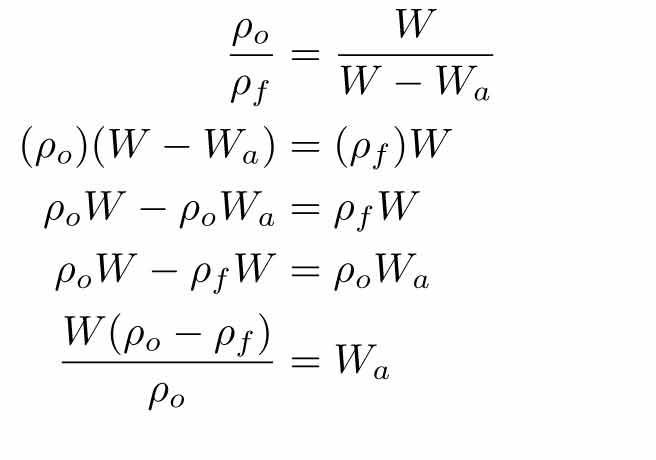SEARCH HOMEMath Central Quandaries & QueriesQuestion from Rob: If an object weighs 100kg in air how much will it weigh when suspended in salt water? Is there an easily used conversion calculation?I think what you mean is what is the difference between the apparent weight of the object in air and in water. Kilograms is a unit of mass and is a measure of the amount of material an object has. Whether the object is in the air or in the water, the amount of material it has will not change so it will still be 100kg. Weight is the force of gravity on an object and is commonly measure in Newtons so the formula for weight is

Weight= mass x acceleration of gravity or W=Fg=mg

When an object is in a fluid like sea water, buoyancy effects the force of gravity so it seems to have a different or apparent weight. If you want to find the apparent weight (Wa), you need to know the density of the object o), the density of the fluid f) and the weight of the object (W):Hope this helps,

JaniceMath Central is supported by the University of Regina and The Pacific Institute for the Mathematical Sciences.